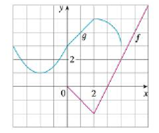Chapter 1.3, Problem 53E### Single Variable Calculus: Early Tr...

8th Edition
James Stewart
ISBN: 9781305270343

#### Solutions

Chapter
Section### Single Variable Calculus: Early Tr...

8th Edition
James Stewart
ISBN: 9781305270343
Textbook Problem

# Use the given graphs of f and g to evaluate each expression, or explain why it is undefined.(a) f(g(2))(b) g(f(0))(c) (f ◦ g)(0)(d) (g ◦ f)(6)(e) (g ◦ g)(−2)(f) (f ◦ f)(4)(a)

To determine

To evaluate: The value of f(g(2)) from the graphs of f and g.

Explanation

From the graph g, the value of g(2) is 5.

Thus, f(g(2))=f(5)

(b)

To determine

To evaluate: The value of g(f(0)) from the graphs of f and g.

(c)

To determine

To find: The value of (fg)(0) from the graphs of f and g.

(d)

To determine

To find: The value of (gf)(6) from the graphs of f and g.

(e)

To determine

To find: The value of (gg)(2) from the graphs of f and g.

(f)

To determine

To find: The value of (ff)(4) from the graphs of f and g.

### Still sussing out bartleby?

Check out a sample textbook solution.

See a sample solution

#### The Solution to Your Study Problems

Bartleby provides explanations to thousands of textbook problems written by our experts, many with advanced degrees!

Get Started

#### Find more solutions based on key concepts